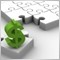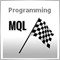How do we plot up arrow and down arrow.974

Hi, can we draw up arrow and down arrow in  an indicator based on only 2 MA cross (for example). Please give an example code of the trick if it can be done.

That is no other indicator.

I include pic to make it clear what I mean.8793

belido:

Hi, can we draw up arrow and down arrow in  an indicator based on only 2 MA cross (for example). Please give an example code of the trick if it can be done.

That is no other indicator.

I include pic to make it clear what I mean.

Hi

If you're using buffers to draw the arrows, just set the buffers to EMPTY_VALUE until you get the cross over.974

Filter:

Hi

If you're using buffers to draw the arrows, just set the buffers to EMPTY_VALUE until you get the cross over.

Do you have idea where to put that EMPTY_VALUE?

Thanks.

StochMain = iStochastic(NULL,0,5,3,3,MODE_SMA,0,MODE_MAIN,0);
StochSignal = iStochastic(NULL,0,5,3,3,MODE_SMA,0,MODE_SIGNAL,0);

if (StochMain > StochSignal )
{
CrossUp[i] = Low[i] - Range;
CrossUp[i] = EMPTY_VALUE;
}
else if (StochMain < StochSignal)
{
CrossDown[i] = High[i] + Range;
CrossDown[i] = EMPTY_VALUE;
}32079

belido:

Hi, can we draw up arrow and down arrow in  an indicator based on only 2 MA cross (for example). Please give an example code of the trick if it can be done.

That is no other indicator.

I include pic to make it clear what I mean.

Hi! If I understand you correctly.

for ( j = limit;  j >= 0;  j -- )
{
CrossUp[j]   = EMPTY_VALUE;
CrossDown[j] = EMPTY_VALUE;
}

for ( i = limit;  i >= 0;  i -- )
{
StochMain    = iStochastic(NULL,0,5,3,3,MODE_SMA,0,MODE_MAIN,i);
StochSignal  = iStochastic(NULL,0,5,3,3,MODE_SMA,0,MODE_SIGNAL,i);
StochMain1   = iStochastic(NULL,0,5,3,3,MODE_SMA,0,MODE_MAIN,i+1);
StochSignal1 = iStochastic(NULL,0,5,3,3,MODE_SMA,0,MODE_SIGNAL,i+1);

if (StochMain >= StochSignal && StochMain1 < StochSignal1)
{
CrossUp[i] = Low[i] - Range;
}
else if (StochMain <= StochSignal && StochMain1 > StochSignal1)
{
CrossDown[i] = High[i] + Range;
}

//----

}

___974

Fillellin:

Hi! If I understand you correctly.

___

That is actually not what I meant. But the the logic is ok. What I mean is how if I want the cross is between StochMain and StochMain1, not between StochMain and StochSignal.

Anyway, thanks for your answer and (I forgot to) thanks also to Filter.